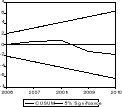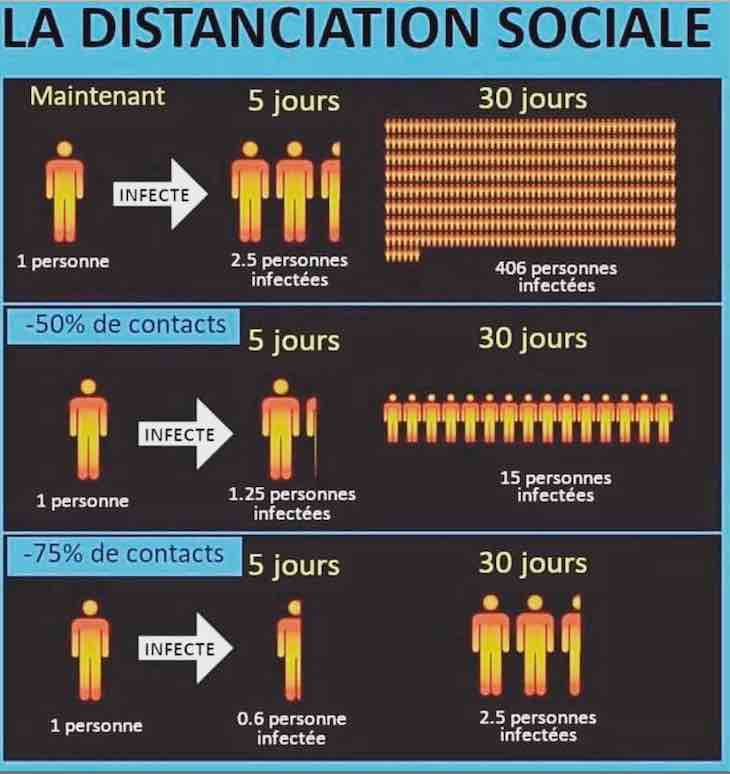WOW !! MUCH LOVE ! SO WORLD PEACE !
Fond bitcoin pour l'amélioration du site: 1memzGeKS7CB3ECNkzSn2qHwxU6NZoJ8o
Dogecoin (tips/pourboires): DCLoo9Dd4qECqpMLurdgGnaoqbftj16NvpRechercher sur le site:

# Tue dole of National Bank of Rwanda from 1995 to 2010

( Télécharger le fichier original )
par Paterne RUKUNDO
National university of Rwanda - A0 2011

## 4.6. Test for stationary

The footstep of this analysis is to determine whether the series are stationary or not. The ADF was used to test for stationary of these series as it provides a superior test to DF, especially in case the residuals of the regression could be serially correlated. The lag length has been automatically selected by AIC from eleven proposed lags and all three possibilities have been tested: neither intercept nor trend, intercept but no trend and both intercept and trend.

TABLE 4.1: Money Stock stationary (MT)

 ADF Test Statistic 5.023678 1% Critical Value* -2.7411 ` ` ` ` 5% Critical Value -1.9658 ` ` ` ` 10% Critical Value -1.6277 *MacKinnon critical values for rejection of hypothesis of a unit root. ` ` ` ` ` ` ` ` ` ` ` ` ` ` ` ` ` ` ` ` Augmented Dickey-Fuller Test Equation Dependent Variable: D(MT) Method: Least Squares Date: 07/20/11 Time: 09:07 Sample(adjusted): 1996 2010 Included observations: 15 after adjusting endpoints Variable Coefficient Std. Error t-Statistic Prob. MT(-1) 0.159544 0.031758 5.023678 0.0002 R-squared 0.322881 Mean dependent var 30.25333 Adjusted R-squared 0.322881 S.D. dependent var 33.05061 S.E. of regression 27.19644 Akaike info criterion 9.508390 Sum squared resid 10355.05 Schwarz criterion 9.555593 Log likelihood -70.31292 Durbin-Watson stat 1.871053

As /ADF/ is greater than /5%/ critical value, MT is stationary at lag 0, level and function of none.

TABLE 4.2: Inflation Gap stationary (IG)

 ADF Test Statistic -4.663015 1% Critical Value* -4.0113 ` ` ` ` 5% Critical Value -3.1003 ` ` ` ` 10% Critical Value -2.6927 *MacKinnon critical values for rejection of hypothesis of a unit root. ` ` ` ` ` ` ` ` ` ` ` ` ` ` ` ` ` ` ` ` Augmented Dickey-Fuller Test Equation Dependent Variable: D(IG) Method: Least Squares Date: 09/19/11 Time: 11:40 Sample(adjusted): 1997 2010 Included observations: 14 after adjusting endpoints Variable Coefficient Std. Error t-Statistic Prob. IG(-1) -2.059676 0.441705 -4.663015 0.0007 D(IG(-1)) 0.347099 0.247617 1.401760 0.1886 C -1.341209 1.262206 -1.062591 0.3107 R-squared 0.796606 Mean dependent var -0.105357 Adjusted R-squared 0.759626 S.D. dependent var 9.320982 S.E. of regression 4.569890 Akaike info criterion 6.064265 Sum squared resid 229.7229 Schwarz criterion 6.201206 Log likelihood -39.44985 F-statistic 21.54115 Durbin-Watson stat 1.603652 Prob(F-statistic) 0.000157

As /ADF/ is greater than all critical values especially /5%/, IG is stationary at lag 1, level and function with intercept.

Table 4.3: Output Gap stationary

 ADF Test Statistic -4.887496 1% Critical Value* -4.1366 ` ` ` ` 5% Critical Value -3.1483 ` ` ` ` 10% Critical Value -2.7180 *MacKinnon critical values for rejection of hypothesis of a unit root. ` ` ` ` ` ` ` ` ` ` ` ` ` ` ` ` ` ` ` ` Augmented Dickey-Fuller Test Equation Dependent Variable: D(YG,3) Method: Least Squares Date: 09/19/11 Time: 12:11 Sample(adjusted): 1999 2010 Included observations: 12 after adjusting endpoints Variable Coefficient Std. Error t-Statistic Prob. D(YG(-1),2) -2.449332 0.501143 -4.887496 0.0009 D(YG(-1),3) 0.595279 0.284900 2.089433 0.0662 C -1.073325 9.702328 -0.110626 0.9143 R-squared 0.832995 Mean dependent var -4.231833 Adjusted R-squared 0.795882 S.D. dependent var 74.27310 S.E. of regression 33.55613 Akaike info criterion 10.07663 Sum squared resid 10134.12 Schwarz criterion 10.19786 Log likelihood -57.45980 F-statistic 22.44524 Durbin-Watson stat 1.843834 Prob(F-statistic) 0.000318

As /ADF/ is greater than all critical values especially /5%/, YG is stationary at lag 1, second difference and function with intercept.

TABLE 4.4: Variation of Exchange stationary

 ADF Test Statistic -4.393650 1% Critical Value -4.1366 ` ` ` ` 5% Critical Value -3.1483 ` ` ` ` 10% Critical Value -2.7180 *MacKinnon critical values for rejection of hypothesis of a unit root. ` ` ` ` ` ` ` ` ` ` ` ` ` ` ` ` ` ` ` ` Augmented Dickey-Fuller Test Equation Dependent Variable: D(DEXCH,3) Method: Least Squares Date: 09/19/11 Time: 12:16 Sample(adjusted): 1999 2010 Included observations: 12 after adjusting endpoints Variable Coefficient Std. Error t-Statistic Prob. D(DEXCH(-1),2) -1.616566 0.367932 -4.393650 0.0013 C 17.89631 23.68642 0.755552 0.4673 R-squared 0.658752 Mean dependent var 14.17417 Adjusted R-squared 0.624627 S.D. dependent var 133.8383 S.E. of regression 81.99966 Akaike info criterion 11.80232 Sum squared resid 67239.43 Schwarz criterion 11.88314 Log likelihood -68.81391 F-statistic 19.30416 Durbin-Watson stat 1.534741 Prob(F-statistic) 0.001348

As /ADF/ is greater than all critical values especially /5%/, DEXCH is stationary at lag 0, second difference and function with intercept.

TABLE 4.5: Previous Money Stock stationary

 ADF Test Statistic -3.699480 1% Critical Value* -4.1366 ` ` ` ` 5% Critical Value -3.1483 ` ` ` ` 10% Critical Value -2.7180 *MacKinnon critical values for rejection of hypothesis of a unit root. ` ` ` ` ` ` ` ` ` ` ` ` ` ` ` ` ` ` ` ` Augmented Dickey-Fuller Test Equation Dependent Variable: D(M1,3) Method: Least Squares Date: 09/19/11 Time: 12:20 Sample(adjusted): 1999 2010 Included observations: 12 after adjusting endpoints Variable Coefficient Std. Error t-Statistic Prob. D(M1(-1),2) -1.159214 0.313345 -3.699480 0.0041 C 0.034242 8.436719 0.004059 0.9968 R-squared 0.577812 Mean dependent var 0.391667 Adjusted R-squared 0.535594 S.D. dependent var 42.88315 S.E. of regression 29.22374 Akaike info criterion 9.738851 Sum squared resid 8540.268 Schwarz criterion 9.819669 Log likelihood -56.43311 F-statistic 13.68615 Durbin-Watson stat 2.076159 Prob(F-statistic) 0.004112

As / ADF/ is greater than / 5%/ critical value, M1 is stationary at lag 0, second difference and function with intercept.

TABLE 4.6: Previous Inflation Gap stationary

 ADF Test Statistic -4.663015 1% Critical Value* -4.0113 ` ` ` ` 5% Critical Value -3.1003 ` ` ` ` 10% Critical Value -2.6927 *MacKinnon critical values for rejection of hypothesis of a unit root. ` ` ` ` ` ` ` ` ` ` ` ` ` ` ` ` ` ` ` ` Augmented Dickey-Fuller Test Equation Dependent Variable: D(IG) Method: Least Squares Date: 09/19/11 Time: 11:40 Sample(adjusted): 1997 2010 Included observations: 14 after adjusting endpoints Variable Coefficient Std. Error t-Statistic Prob. IG(-1) -2.059676 0.441705 -4.663015 0.0007 D(IG(-1)) 0.347099 0.247617 1.401760 0.1886 C -1.341209 1.262206 -1.062591 0.3107 R-squared 0.796606 Mean dependent var 0.105357 Adjusted R-squared 0.759626 S.D. dependent var 9.320982 S.E. of regression 4.569890 Akaike info criterion 6.064265 Sum squared resid 229.7229 Schwarz criterion 6.201206 Log likelihood -39.44985 F-statistic 21.54115 Durbin-Watson stat 1.603652 Prob(F-statistic) 0.000157

As /ADF/ is greater than all critical values especially /5%/, IG1 is stationary at lag 1, level and function with intercept.

Table 4.7: previous Output Gap stationary

 ADF Test Statistic -5.810852 1% Critical Value* -4.1366 ` ` ` ` 5% Critical Value -3.1483 ` ` ` ` 10% Critical Value -2.7180 *MacKinnon critical values for rejection of hypothesis of a unit root. ` ` ` ` ` ` ` ` ` ` ` ` ` ` ` ` ` ` ` ` Augmented Dickey-Fuller Test Equation Dependent Variable: D(YG1,3) Method: Least Squares Date: 09/19/11 Time: 12:27 Sample(adjusted): 1999 2010 Included observations: 12 after adjusting endpoints Variable Coefficient Std. Error t-Statistic Prob. D(YG1(-1),2) -1.539314 0.264903 -5.810852 0.0002 C 1.842228 10.71345 0.171955 0.8669 R-squared 0.771512 Mean dependent var 0.864250 Adjusted R-squared 0.748663 S.D. dependent var 74.01819 S.E. of regression 37.10790 Akaike info criterion 10.21655 Sum squared resid 13769.96 Schwarz criterion 10.29737 Log likelihood -59.29929 F-statistic 33.76600 Durbin-Watson stat 2.583075 Prob(F-statistic) 0.000170

As /ADF/ is greater than all critical values especially /5%/, YG1 is stationary at lag 0, second difference and function with intercept.

Table 4.8: Previous Variation of Exchange stationary

 ADF Test Statistic -5.187147 1% Critical Value* -4.1366 ` ` ` ` 5% Critical Value -3.1483 ` ` ` ` 10% Critical Value -2.7180 *MacKinnon critical values for rejection of hypothesis of a unit root. ` ` ` ` ` ` ` ` ` ` ` ` ` ` ` ` ` ` ` ` Augmented Dickey-Fuller Test Equation Dependent Variable: D(DEXCH1,2) Method: Least Squares Date: 09/19/11 Time: 12:36 Sample(adjusted): 1999 2010 Included observations: 12 after adjusting endpoints Variable Coefficient Std. Error t-Statistic Prob. D(DEXCH1(-1)) -1.469962 0.283386 -5.187147 0.0004 C 1.485446 10.59132 0.140251 0.8912 R-squared 0.729045 Mean dependent var 2.302500 Adjusted R-squared 0.701949 S.D. dependent var 67.19665 S.E. of regression 36.68534 Akaike info criterion 10.19364 Sum squared resid 13458.14 Schwarz criterion 10.27446 Log likelihood -59.16186 F-statistic 26.90649 Durbin-Watson stat 2.085680 Prob(F-statistic) 0.000409

As /ADF/ is greater than all critical value /5%/, DEXCH1 is stationary at lag 0, first difference and function with intercept.

Table 4.9: Error Term stationary

 ADF Test Statistic -4.335477 1% Critical Value* -4.1366 ` ` ` ` 5% Critical Value -3.1483 ` ` ` ` 10% Critical Value -2.7180 *MacKinnon critical values for rejection of hypothesis of a unit root. ` ` ` ` ` ` ` ` ` ` ` ` ` ` ` ` ` ` ` ` Augmented Dickey-Fuller Test Equation Dependent Variable: D(E) Method: Least Squares Date: 07/20/11 Time: 09:46 Sample(adjusted): 1999 2010 Included observations: 12 after adjusting endpoints Variable Coefficient Std. Error t-Statistic Prob. E(-1) -1.299467 0.299729 -4.335477 0.0015 C 16.62786 9.471546 1.755559 0.1097 R-squared 0.652734 Mean dependent var 1.986182 Adjusted R-squared 0.618007 S.D. dependent var 49.59723 S.E. of regression 30.65385 Akaike info criterion 9.834405 Sum squared resid 9396.583 Schwarz criterion 9.915223 Log likelihood -57.00643 F-statistic 18.79636 Durbin-Watson stat 1.976710 Prob(F-statistic) 0.001477

As /ADF/ is greater than /5%/ critical value, error term is stationary at 0 lag, level and function with intercept.

Notes: all tests of stationary are done in Eviews 3.1

CUSUM TESTSource of basic data: BNR, NISR and MINECOFIN

As the line of cusum does not get out of the corridor, means that the parameters of the regression model are stable.

M = 48.62344 + 0.996100M1 + 1.492988IG + 2.824734IG1 - 0.549954YG + 0.099609YG1 -

P (0.001) (0.003) (0.002) (0.000) (0.02) (0.007)

0.088485EX - 0.311933EX1.

(0.0045) (0.01)

F table = 4.7 Fcritical = 38.02

R2 = 0.98 dw = 2.46

As dw is greater than R2, the estimated model is correctly specified.

DW and BREUCH-GODFREY TEST show that there is no autocorrelation because the

DW is the zone of none autocorrelation zone and nR2 > ÷2 for LM (Lagrangian Multiplier or Breuch-Godfrey Test)

nR2=31.36 > X2 =9.88623

R2 is coming from estimation of errors.

All coefficients have an econometric sense explained by Fcritical that is greater than Ftable , means that the concerned variables have an effect on current money stock aggregate.

After testing stationary for all variables and model specification, now interpretation.